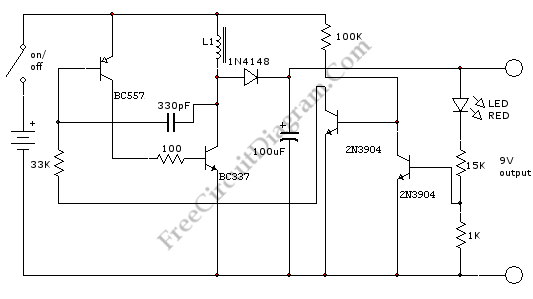# Small DC-DC Inverter: Convert 1.5 to 9 voltsThis is a 1.5 to 9 volts inverter schematic circuit. This is low cost and a simple circuit that need few components.This circuit is used in many application that requires 9 volts power supply. using this circuit we can replace the expensive 9v batteries. This circuit can convert 1.5V to 9V. This circuit can be used only for application that need low current. Here is the circuit:This circuit requires 1.5 volts up to 4.5 volts input. The current of this circuit is less than 10mA when no current that is drawn from the output. This circuit use inductor L1 that must have a 0.25 mm diameter enameled wire, and 60 turns on a 10 mm ferrite slug 15 mm long.